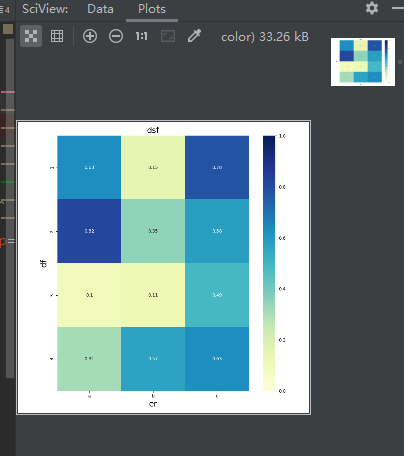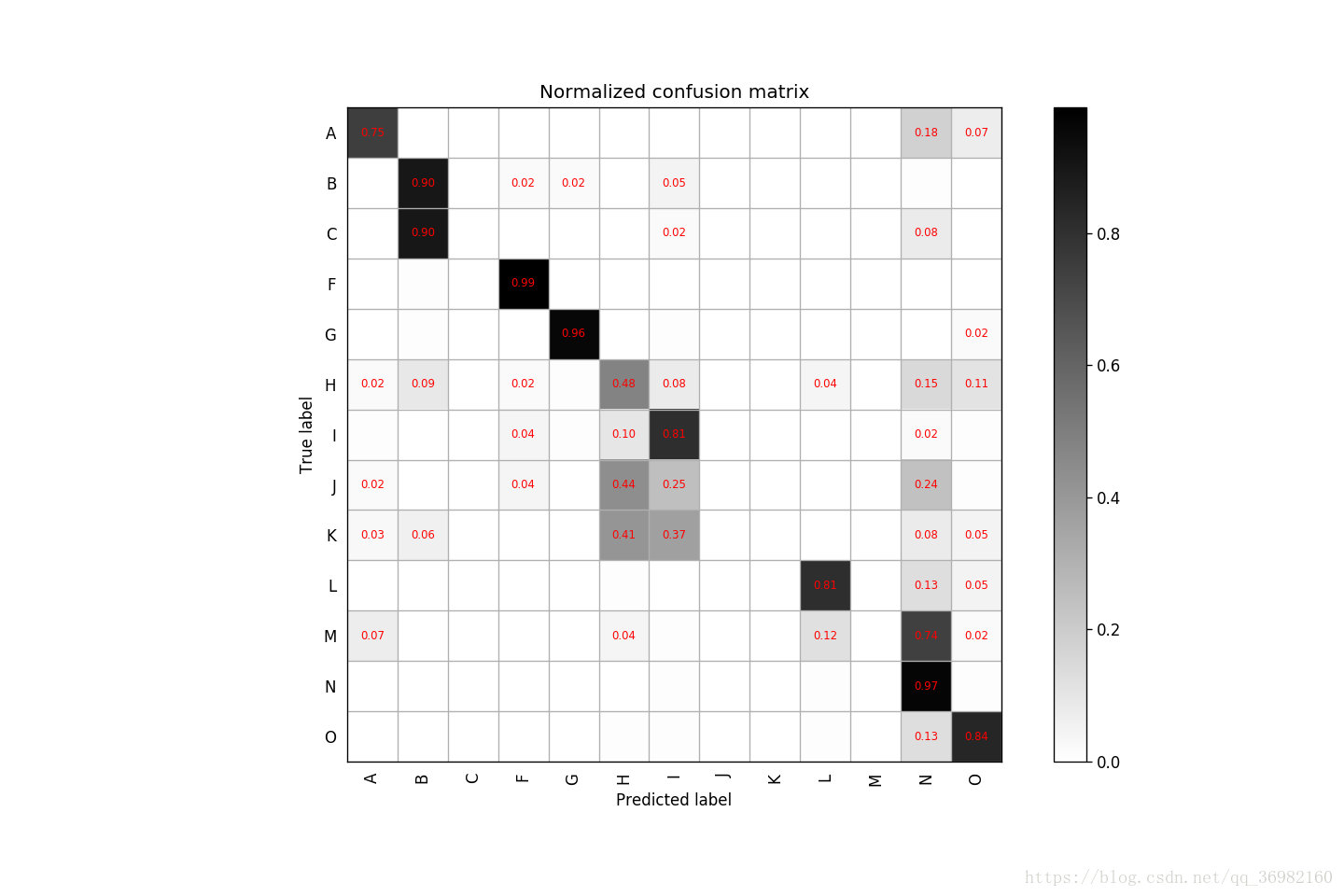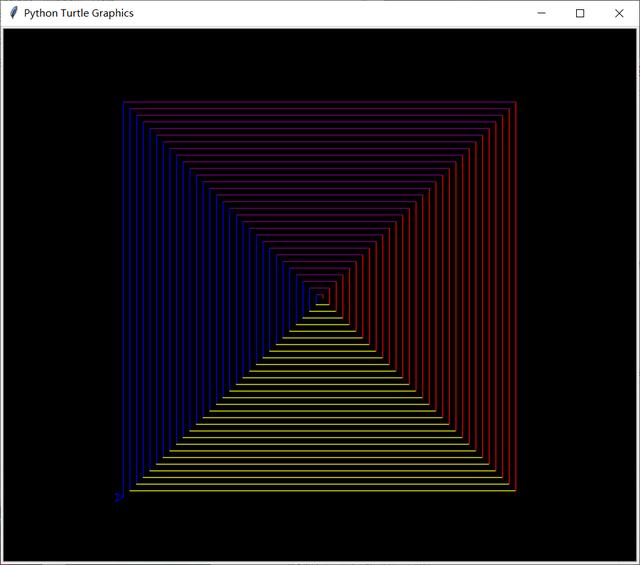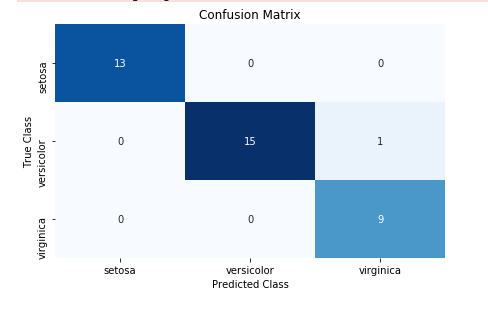• ## python绘制矩阵权重

千次阅读 2020-06-14 15:17:04
如果是整数n，则绘制列名，但每个n绘制一个label。 默认为True。 yticklabels: 如果是True，则绘制dataframe的行名。如果是False，则不绘制行名。如果是列表，则绘制列表中的内容作为yticklabels。 如果是整数n，则...
参考：https://blog.csdn.net/henbile/article/details/80241597
https://blog.csdn.net/m0_38103546/article/details/79935671
import matplotlib.pyplot as plt
import seaborn as sns
import numpy as np
import pandas as pd
a = np.random.rand(4,3)
fig, ax = plt.subplots(figsize=(9,9))
#二维的数组的热力图，横轴和数轴的ticklabels要加上去的话，既可以通过将array转换成有column
#和index的DataFrame直接绘图生成，也可以后续再加上去。后面加上去的话，更灵活，包括可设置labels大小方向等。
sns.heatmap(pd.DataFrame(np.round(a,2), columns=['a', 'b', 'c'], index=range(1,5)),
annot=True, vmax=1,vmin=0, xticklabels=True, yticklabels=True, square=True, cmap="YlGnBu")
#sns.heatmap(np.round(a,2), annot=True, vmax=1,vmin = 0, xticklabels= True, yticklabels= True,
#            square=True, cmap="YlGnBu")
ax.set_title('dsf', fontsize=18)
ax.set_ylabel('df', fontsize=18)
ax.set_xlabel('er', fontsize=18)

plt.show()seaborn.heatmap()各个参数
seaborn.heatmap(data, vmin=None, vmax=None, cmap=None, center=None, robust=False, annot=None, fmt='.2g', annotkws=None, linewidths=0, linecolor='white', cbar=True, cbarkws=None, cbar_ax=None, square=False, ax=None, xticklabels=True, yticklabels=True, mask=None, **kwargs)
data：矩阵数据集，可以使numpy的数组（array），如果是pandas的dataframe，则df的index/column信息会分别对应到heatmap的columns和rowslinewidths,热力图矩阵之间的间隔大小vmax,vmin, 图例中最大值和最小值的显示值，没有该参数时默认不显示cmap：matplotlib的colormap名称或颜色对象；如果没有提供，默认为cubehelix map (数据集为连续数据集时) 或 RdBu_r (数据集为离散数据集时)center:将数据设置为图例中的均值数据，即图例中心的数据值；通过设置center值，可以调整生成的图像颜色的整体深浅；设置center数据时，如果有数据溢出，则手动设置的vmax、vmin会自动改变xticklabels: 如果是True，则绘制dataframe的列名。如果是False，则不绘制列名。如果是列表，则绘制列表中的内容作为xticklabels。 如果是整数n，则绘制列名，但每个n绘制一个label。 默认为True。yticklabels: 如果是True，则绘制dataframe的行名。如果是False，则不绘制行名。如果是列表，则绘制列表中的内容作为yticklabels。 如果是整数n，则绘制列名，但每个n绘制一个label。 默认为True。默认为True。anot: annotate的缩写，annot默认为False，当annot为True时，在heatmap中每个方格写入数据

展开全文• 本篇文章小编给大家介绍Python彩色螺旋矩阵绘制，在文章中小编详细的解析了绘值螺旋矩形的代码，对于“懒癌”小伙伴们，小编还给大家提供了完整的代码文件，可直接运行。
• 涉及到分类问题，我们经常需要通过可视化混淆矩阵来分析实验结果进而得出调参思路，本文介绍如何利用python绘制混淆矩阵（confusion_matrix），本文只提供代码，给出必要注释。 Code # -*-coding:utf-8-*- from ...
Summary
涉及到分类问题，我们经常需要通过可视化混淆矩阵来分析实验结果进而得出调参思路，本文介绍如何利用python绘制混淆矩阵（confusion_matrix），本文只提供代码，给出必要注释。
Code​
# -*-coding:utf-8-*-
from sklearn.metrics import confusion_matrix
import matplotlib.pyplot as plt
import numpy as np

#labels表示你不同类别的代号，比如这里的demo中有13个类别
labels = ['A', 'B', 'C', 'F', 'G', 'H', 'I', 'J', 'K', 'L', 'M', 'N', 'O']

'''
具体解释一下re_label.txt和pr_label.txt这两个文件，比如你有100个样本
去做预测，这100个样本中一共有10类，那么首先这100个样本的真实label你一定
是知道的，一共有10个类别，用[0,9]表示，则re_label.txt文件中应该有100
个数字，第n个数字代表的是第n个样本的真实label（100个样本自然就有100个
数字）。
同理，pr_label.txt里面也应该有1--个数字，第n个数字代表的是第n个样本经过
你训练好的网络预测出来的预测label。
这样，re_label.txt和pr_label.txt这两个文件分别代表了你样本的真实label和预测label，然后读到y_true和y_pred这两个变量中计算后面的混淆矩阵。当然，不一定非要使用这种txt格式的文件读入的方式，只要你最后将你的真实
label和预测label分别保存到y_true和y_pred这两个变量中即可。
'''

tick_marks = np.array(range(len(labels))) + 0.5

def plot_confusion_matrix(cm, title='Confusion Matrix', cmap=plt.cm.binary):
plt.imshow(cm, interpolation='nearest', cmap=cmap)
plt.title(title)
plt.colorbar()
xlocations = np.array(range(len(labels)))
plt.xticks(xlocations, labels, rotation=90)
plt.yticks(xlocations, labels)
plt.ylabel('True label')
plt.xlabel('Predicted label')

cm = confusion_matrix(y_true, y_pred)
np.set_printoptions(precision=2)
cm_normalized = cm.astype('float') / cm.sum(axis=1)[:, np.newaxis]
print cm_normalized
plt.figure(figsize=(12, 8), dpi=120)

ind_array = np.arange(len(labels))
x, y = np.meshgrid(ind_array, ind_array)

for x_val, y_val in zip(x.flatten(), y.flatten()):
c = cm_normalized[y_val][x_val]
if c > 0.01:
plt.text(x_val, y_val, "%0.2f" % (c,), color='red', fontsize=7, va='center', ha='center')
# offset the tick
plt.gca().set_xticks(tick_marks, minor=True)
plt.gca().set_yticks(tick_marks, minor=True)
plt.gca().xaxis.set_ticks_position('none')
plt.gca().yaxis.set_ticks_position('none')
plt.grid(True, which='minor', linestyle='-')

plot_confusion_matrix(cm_normalized, title='Normalized confusion matrix')
# show confusion matrix
plt.savefig('../Data/confusion_matrix.png', format='png')
plt.show()


ResultInstructions
按照代码中的注释将labels、y_true 、y_pred替换为你自己的数据即可。
Reference
如何用python画好confusion matrix 
展开全文可视化 深度学习
• 本文章小编教大家使用Python海龟绘制彩色螺旋矩阵，下面是相关代码块的解析，仅供喜爱python的小伙伴们学习参考。1. 导入turtle库from turtle import*2.设置画布背景，将背景设置为黑色bgcolor("black")3.设置画笔...

本文章小编教大家使用Python海龟绘制彩色螺旋矩阵，下面是相关代码块的解析，仅供喜爱python的小伙伴们学习参考。
1. 导入turtle库
from turtle import*
2.设置画布背景，将背景设置为黑色
bgcolor("black")
3.设置画笔绘制速度
speed(0)
4.定义变量colors用于存放颜色，颜色可根据个人喜好选择
colors=["yellow","red","purple","blue"]
5.自定义函数，用于定义功能，小编在这里简化绘制矩形的代码，使用循环语句，起到精简程序的作用
def square(m):
for i in range(m):
pencolor(colors[i%4])
forward(i*4)
left(90)
6.调用函数，在这里函数的参数值可以修改，数值越大，绘制出来的矩阵越大
square(360)
最后运行出来的彩色螺旋矩阵如下图：小琳爱学习

好了，小编就分享到这里，彩色螺旋矩阵就绘制完成了！创作不易，点个赞打个赏再走吧！完整的代码可参见链接：链接：https://pan.baidu.com/s/1ZWlDmHYmKKxF7Fi0uy0aKQ
提取码：s6x6
复制这段内容后打开百度网盘手机App，操作更方便哦
想学更多的Python内容吗？学习更多内容请关注小琳的公众号，和小琳一起悄悄拔尖儿！展开全文• 主要介绍了详解使用python绘制混淆矩阵（confusion_matrix），文中通过示例代码介绍的非常详细，对大家的学习或者工作具有一定的参考学习价值，需要的朋友们下面随着小编来一起学习学习吧
• 使用python绘制混淆矩阵 # 可视化分类器性能 # load libraries import matplotlib.pyplot as plt import seaborn as sns from sklearn import datasets from sklearn.linear_model import LogisticRegression from ...
使用python绘制混淆矩阵
# 可视化分类器性能
import matplotlib.pyplot as plt
import seaborn as sns
from sklearn import datasets
from sklearn.linear_model import LogisticRegression
from sklearn.model_selection import train_test_split
from sklearn.metrics import confusion_matrix
import pandas as pd

# create feature matrix
features = iris.data

# create target vector
target = iris.target

# create list of target class names
class_names = iris.target_names

# split into training and test sets
# 创建 训练集 测试集
features_train, features_test, target_train, target_test = train_test_split(features, target, random_state=1)

# create logistic regression 逻辑回归
classifier = LogisticRegression()

# train model and make predictions 做出预测
target_predicted = classifier.fit(features_train, target_train).predict(features_test)

# create confusion matrix 创建混淆矩阵
matrix = confusion_matrix(target_test, target_predicted)

# create pandas dataframe   创建数据集
dataframe = pd.DataFrame(matrix, index=class_names, columns=class_names)

# create heatmap 绘制热力图
sns.heatmap(dataframe, annot=True, cbar=None, cmap="Blues")
plt.title("Confusion Matrix"), plt.tight_layout()
plt.ylabel("True Class"), plt.xlabel("Predicted Class")
plt.show()展开全文• Python 绘制混淆矩阵函数 confusion_matrix代码如下：def plot_confusion_matrix(y_true, y_pred, labels): import matplotlib.pyplot as plt from sklearn.metrics import confusion_matrix cmap = plt.cm....confusion matrix
• 文章目录使用python绘制混淆矩阵（可直接复制调用） 使用python绘制混淆矩阵（可直接复制调用） 转自https://www.jianshu.com/p/13debf42fdb7 import matplotlib.pyplot as plt from sklearn.metrics import ...
• Python绘制彩色螺旋矩阵 本文章小编教大家使用Python海龟绘制彩色螺旋矩阵，下面是相关代码块的解析，仅供喜爱python的小伙伴们学习参考。 1. 导入turtle库 from turtle import* 2.设置画布背景，将背景设置为...编程语言 人工智能 机器学习 深度学习
• 主要介绍了Python+pillow绘制矩阵盖尔圆简单实例，具有一定借鉴价值,需要的朋友可以参考下
• 数据科学最重要的技能之一就是...这里简单汇总一下使用Python绘制传统相关性矩阵/下三角相关性矩阵/重点相关性矩阵的代码 1.传统相关性矩阵 import pandas as pd import seaborn as sns import matplotlib.pyp...
• 1. 在计算出混淆矩阵之后，想自己绘制图形（并且在图形上显示汉字），可用 #coding=utf-8 import matplotlib.pyplot as plt import numpy as np confusion = np.array(([91,0,0],[0,92,1],[0,0,95])) # 热度图，...
• 一、plt.cm绘制 示例一：设置0-100的10*10的矩阵 代码1： import matplotlib.pyplot as plt import numpy as np A = np.arange(0, 100).reshape(10, 10) plt.matshow(mat, cmap=plt.cm.Reds)#这里设置颜色为...
• 话不多说，直接上题 @lke 说：你好，我运行了这段代码，我想得到的是一个三维矩阵，可是输出的结果却是矩阵里面还包含了一个数组。 我的思路是将t1与t2连接起来，然后循环赋值给input数组，请问有什么问题吗？ 输出...
• python是拿list表示数组的，毕竟不是矩阵（matrix）实验室（laboratory）嘛2333python直接复制格式标准的数据是可以识别成list的，但我要是输入一个规整的矩阵就繁琐了些。 比如这种1 4 7 102 5 8 113 6 9 12 python...
• v2, v3]).T # 绘制散点图矩阵 pd.plotting.scatter_matrix(df) plt.show() 图形 2、示例 2 代码import numpy as np import pandas as pd import matplotlib.pyplot as plt # 生成数据 v1 = np.random.normal(0, 1, ...
• import numpy as np import matplotlib.pyplot as plt from pylab import * conf_arr=[[13,0,0,0,0,1,0,0,0,0,0,0,1,0,0,0,2,0,0,0,0,0,0,0,0,0,0,0,1,0,0,0,0,0,0,0,0,1,0,0,1,0,0,0,4,0,5,0,1,0,0,], ...
• 本文介绍python中seaborn.pairplot和seaborn.PairGrid绘制矩阵数据可视化 数据挖掘 机器学习 大数据
• 在使用Python绘制图表前，我们需要先安装两个库文件numpy和matplotlib。Numpy是Python开源的数值计算扩展，可用来存储和处理大型矩阵，比Python自身数据结构要高效；matplotlib是一个Python的图像框架，使用其绘制...
• python +gis 点数据转栅格数据 绘制矩阵图 import numpy as np import pandas as pd import matplotlib.pyplot as plt import matplotlib as mpl mpl.rcParams['font.sans-serif'] = ['SimHei'] #将全局字体设...
• plt.imshow(confusion_matrix, interpolation='nearest', cmap=plt.cm.Oranges) #按照像素显示出矩阵 plt.title('confusion_matrix') plt.colorbar() tick_marks = np.arange(len(classes)) plt.xticks(tick_marks, ...
• heatmap要求：绘制200200的压缩矩阵，剔除边角料（如423856的矩阵画出来实际上只用到了前400*800的数据，多余的数据丢弃。因为我的矩阵很大的缘故，这样的数据取舍不影响整体观察）。在heatmap中画出行列比。 一次性...
• 本文主要讲解如何使用python绘制三维的柱形图，如下图源代码如下：1234567891011121314151617181920212223242526272829import numpy as npimport matplotlib.pyplot as pltfrom mpl_toolkits.mplot3dimport Axes3D#...
• python绘制函数图像代码，NumPy(Numerical Python) 是 Python 语言的一个扩展程序库，支持大量的维度数组与矩阵运算，此外也针对数组运算提供大量的数学函数库。 NumPy 的前身 Numeric 最早是由 Jim Hugunin 与其它......

# python绘制矩阵python 订阅